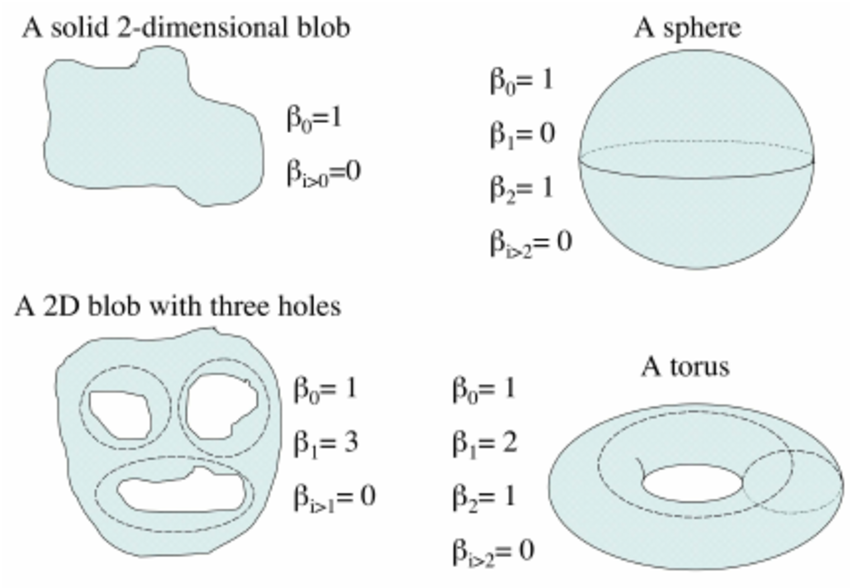# Topological Deep Learning & Machine Learning

Source: Deep Learning on Medium# What has happened so far?

We explained that Topological Data Analysis is Superior to Pixel Based Methods like Deep Learning…

Then we went through some theory behind Topological Data Analysis and saw some examples using the toolkit Scikit-tda…

And then finally we saw some quick examples with a toolkit called Gudhi…

and now here we are…

• Topology + Machine learning
• Topology + Deep Learning

And the most important thing is to structure our thoughts and not go all over the place. So that we can make a clear and crisp use case for integrating topology with machine learning and deep learning. And there is no confusion as to how to go about doing it.

# And how do we start doing that? We start with e.g. Betti Numbers.

I’m sure you would have gone through the previous articles and understand what are betti numbers. But to summarize again…

Betti numbers are a series of numbers that describe a shape.

For the purposes of this article, we don’t need to know anything more than — every different shape will have a different set of betti numbers b0-bn where n is the nth betti number.

To integrate Topology we will feed betti numbers into our backend Machine Learning and Deep Learning algorithms.

And we will also assume that our shapes are going to be only so complex so we will only feed the first ’n’ betti numbers e.g. 5 betti numbers corresponding to our shapes e.g. b0, b1, b2, b3, b4 only.

And we also know that since betti numbers count the number of holes in nth dimension. And there is no limit to the number of holes. We will assume that the max number of holes we will consider (max complexity of our shapes) will be for example 10.

So each of the betti numbers b0, b1, b2, b3, b4 can be from 0–10 both inclusive.

Now we also understand that we have to fit these numbers appropriately into our backend Machine Learning and Deep Learning numbers. We understand that some backend algorithms would be able to process a betti number between 0–10 as is. But deep learning would take a number between 0.0–1.0 so we will scale our betti numbers (normalize their values) from 0.0–1.0 so 0 will be 0.0, 1 will be 0.1, 2 will be 0.2, 10 will be 1.0.

So what is the big idea?

• Betti numbers describe shapes
• If we can feed betti numbers into our backend Machine Learning / Deep Learning algorithms we will be able to create algorithms that learn something based on shapes (and not pixel image data)
• That should (would) be superior to all our Image Pixel based Machine Learning and Deep Learning Algorithms we have been building so far.